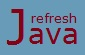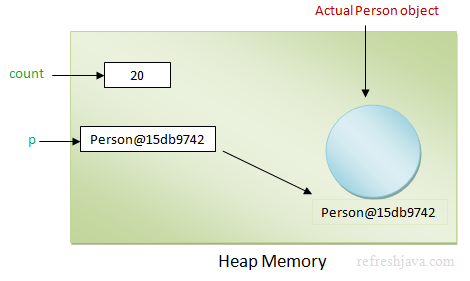# Call by Value in Java

Call by value and call by reference is a mechanism of passing argument while calling a method. When we pass an argument to a method while calling, it is passed using either call by value or call by reference mechanism. Since java uses only call by value mechanism, so we will discuss only call by value mechanism in this tutorial. Java doesn't uses call by reference mechanism in any way for passing argument to methods.

Before we understand call by value mechanism, let's first understand how a variable or object is stored in java, it will help us to understand the mechanism better.

In java all primitive types are value types which means all primitive variables in java stores it's value directly inside memory. While all non primitive types are reference types which means non primitive variables stores the references, not the value(object) directly. The actual object in java is created somewhere else in memory, the reference of this object is stored as value in non primitive variable. Let's understand this by an example and image below to clarify the things properly. Let's consider `Person` is a class.

``` `int` count = 20;
Person p = `new` Person();
```As you can see the value of variable `count` is stored directly. If you access the `count` in your program, it's the value that will be returned. While variable `p` holds the reference of actual object as value, the actual `Person` object is created somewhere else. So if you access the non primitive variable, it's the reference of actual object that will be returned not the actual object itself.

Note : The reference(`Person@15db9742`) of object `p` given in above image is not the exact memory address. It is just used as an example to understand how non-primitive variables are stored in java.

## How parameters are passed in Java

In java whenever you are passing a primitive type variable, it's the copy of variable value that is passed to called method. This value is now referred by corresponding called method parameter. So if you make any changes in that parameter value, it won't be reflected in calling method. While when we pass reference type variable, it's the copy of reference that is passed to called method. Since this reference points to an actual object, if you make any changes using this reference, the changes will be reflected in both the methods, calling and called methods.

### How references are passed in java ?

In java, the reference of an object is itself a value. In call by value mechanism, this value(reference) is itself passed to called method.

### Call by value program in Java

The program below shows the call by value mechanism for primitive type variables in java. The changes made in called method will not be reflected in calling method.

```` class` CallByValue {
`static int` a = 20;
`public static void` main(String [] args) {
`int` b = 30;
System.out.println(`"Before method call, a = "`+a+ `", b = "`+b);
changeValues(a,b);
System.out.println(`"After method call, a = "`+a+ `", b = "`+b);
}
`public static void` changeValues(`int` p, `int` q) {
p = 40;
q = 50;
System.out.println(`"In changeValues method, a = " `+a+ `", p = "`+p+`", q = "`+q);
}
}```

Output:

Before method call, a = 20, b = 30
In changeValues method, a = 20, p = 40, q = 50
After method call, a = 20, b = 30

As you can see from the output, even after changing the values in `changeValues` method, the value of variable `a` and `b` is still remain same in `main` method, because it's the copy of value of variable `a` and `b` which is passed to called method.

The program below shows the call by value mechanism for reference type variables. The changes made in called method would be reflected in calling method as well.

```` class` Person {
`int` age;
`int` height;

`public` Person(`int` a, `int` h) {
age = a;
height = h;
}
`public static void` main(String [] args) {
Person p = `new` Person(20,170);
System.out.println(`"Before method call, age = "`+p.age+ `", height = "`+p.height);
changeValues(p);
System.out.println(`"After method call, age = "`+p.age+ `", height = "`+p.height);
}
`public static void` changeValues(Person q) {
q.age = 30;
q.height = 180;
System.out.println(`"In changeValues method, age = "`+q.age+ `", height = "`+q.height);
}
}```

Output:

Before method call, age = 20, height = 170
In changeValues method, age = 30, height = 180
After method call, age = 30, height = 180

As you can see from the output, after changing the values in `changeValues` method, the value of attributes `age` and `height` for object `p` is changed. This is happening because the reference of object `p` is passed to method `changeValues` which is then assigned in object `q`. Object `q` makes the changes on same reference that is why the changes are reflected in calling(`main`) method as well.

If you assign `null` or create and assign a new object(`new Person(30,160)`) to object `q` in above example, the actual object `p` will not be affected, it will still be pointing to same object.

## How String variables are passed in Java

Since `String` is also a non primitive data type in java, so it's the reference of variable which is passed to called method. But the interesting thing is that, if you make any changes in `String` variable in called method, it doesn't reflects in calling method. Let's see the example first, then we will understand the reason behind this.

```` class` CallByValueForString {
`public static void` main(String [] args) {
String str = `"String"`;
StringBuffer strbuf = `new` StringBuffer(`"StringBuffer")`;
System.out.println(`"Before method call, str = "`+str+ `", strbuf = "`+strbuf);
changeValue(str,strbuf);
System.out.println(`"After method call, str = "`+str+ `", strbuf = "`+strbuf);
}
`public static void` changeValue(String temp, StringBuffer tempbuf) {
temp = `"String Modified"`;
tempbuf = tempbuf.append(`" Modified"`);
System.out.println(`"In changeValue method, temp = "`+temp+ `", tempbuf = "`+tempbuf);
}
}```

Output:

Before method call, str = String, strbuf = StringBuffer
In changeValue method, temp = String Modified, tempbuf = StringBuffer Modified
After method call, str = String, strbuf = StringBuffer Modified

As you can see from the output, the value of `String` variable `str` doesn't change even after method call while the value of `StringBuffer` variable `strbuf` get's changed after method call. This is because `String` in java is `immutable` while `StringBuffer` is `mutable`. If you make any changes in `String` variable, a new string is created inside the string pool, the original string still remains same that is why the value of `str` doesn't change even after method call.

### What if I pass objects of wrapper classes like Integer, Float, Character etc in method calling ?

Wrapper classes in java are also immutable like `String` class, if you make any changes in called method, it won't be reflected in calling method.

★★★
• call by value and pass by value are same thing.
• Actual object itself is never passed, it's the reference of actual object which is passed to called method.
• You can change only state/attribute of object using the reference in called method.
• In C/C++, the reference directly points to the memory address of a variable.
• If you are passing expressions like a+b, a*b etc, it's the final evaluated value of expression that will be passed to called method.